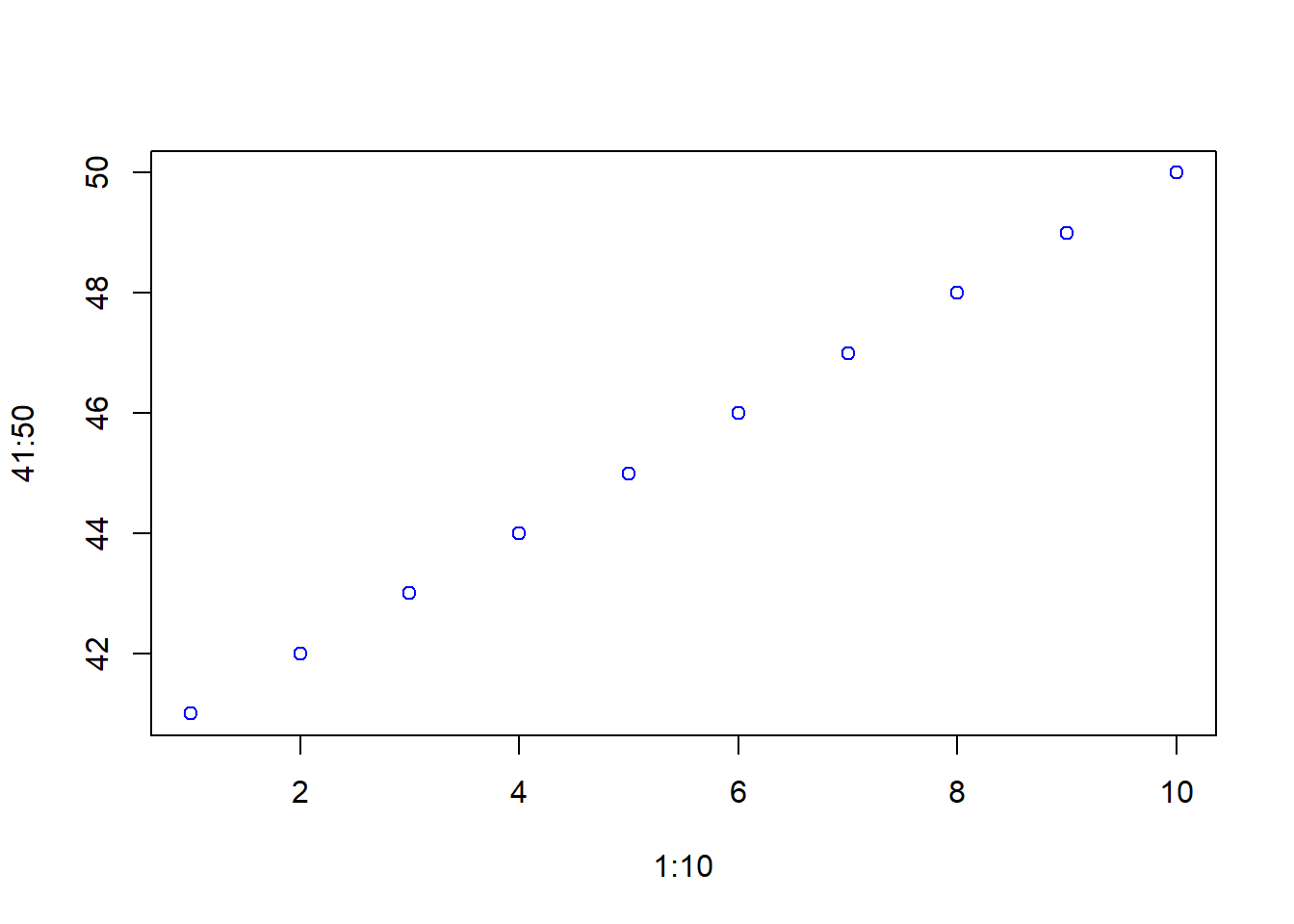# Hello World: Blogging using Rmd

This is the Hello World blog post using Rmd…

Let’s try some math:

$\beta = \alpha$

How about inline math such as $b + \alpha$

plot(1:10, 41:50, col = "blue")Figure 1: This is a simple plot

Let’s try mentioning r function.

plot()

This is an alert. R function within the alert: sum()

Do not get serious with arrange()
blogdown::install_hugo()
install_hugo()